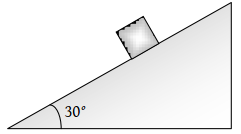The time taken by a block of wood (initially at rest) to slide down a smooth inclined plane 9.8 m long (angle of inclination is ${30}^{o}$) is(1) $\frac{1}{2}\mathrm{sec}$

(2) 2 sec

(3) 4 sec

(4) 1 sec

Concept Videos :-

#26-Equations-for-Uniform-Acceleration
#27-Equations for Uniform Acceleration 28Calculus Method29
#28-Sign Convention
#29-Solved Examples 16
#31-Solved-Examples-18
#32-Solved Examples 19
#33-Solved Examples 20
#34-Solved-Examples-21
#35 Solved Examples 22
#30-Solved Examples 17

Concept Questions :-

Uniformly accelerated motion

(2) For one dimensional motion along a plane

$S=ut+\frac{1}{2}a{t}^{2}⇒9.8=O+\frac{1}{2}g\mathrm{sin}{30}^{o}{t}^{2}$$t=2\mathrm{sec}$

Difficulty Level:

• 13%
• 70%
• 10%
• 10%